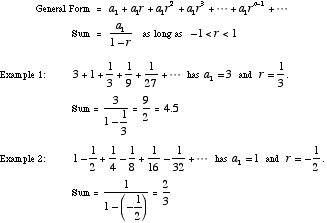infinite geometric series

Infinite Geometric Series

An infinite series that is geometric. An infinite geometric series converges if its common ratiorsatisfies –1 < r < 1. Otherwise it diverges.